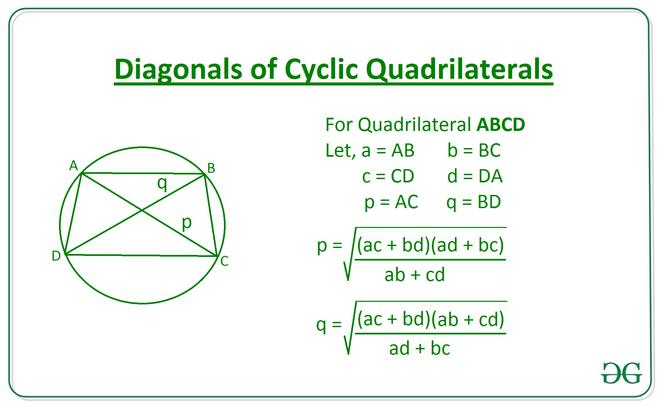Related Articles
Length of Diagonals of a Cyclic Quadrilateral using the length of Sides.
• Last Updated : 22 Apr, 2021

Given integers A, B, C, and D, denoting the length of sides of a Cyclic Quadrilateral, the task is to find the length of diagonals of a cyclic quadrilateral.

Examples:

Input: A = 10, B = 15, C = 20, D = 25
Output: 22.06 26.07
Input: A = 10, B = 30, C =50, D = 20
Output: 37.93 29.0

Approach: The length of diagonals can be calculated using the following equations:[Tex]Diagonal (q)=\sqrt{\frac{(ac+bd)(ab+cd)}{ad+bc}}          [/Tex]Below is the implementation of the above approach:

## C++

 // C++ Program to implement// the above approach#include using namespace std; // Function to calcualte the length of// diagonals of a cyclic quadrilateralvector<float> Diagonals(int a, int b,                        int c, int d){    vector<float> ans;    ans.push_back(sqrt(((a * c) + (b * d)) *                       ((a * d) + (b * c)) /                       ((a * b) + (c * d))));    ans.push_back(sqrt(((a * c) + (b * d)) *                       ((a * b) + (c * d)) /                       ((a * d) + (b * c))));    return ans;} // Driver Codeint main(){    int A = 10;    int B = 15;    int C = 20;    int D = 25;     // Function Call    vector<float> ans = Diagonals(A, B, C, D);     // Print the final answer    printf("%.2f %.2f",           (ans) + .01,            ans + .01);} // This code is contributed by Amit Katiyar

## Java

 // Java Program to implement// the above approachimport java.util.*;class GFG{ // Function to calcualte the length of// diagonals of a cyclic quadrilateralstatic Vector Diagonals(int a, int b,                               int c, int d){    Vector ans = new Vector();    ans.add((float) Math.sqrt(((a * c) + (b * d)) *                              ((a * d) + (b * c)) /                              ((a * b) + (c * d))));    ans.add((float) Math.sqrt(((a * c) + (b * d)) *                              ((a * b) + (c * d)) /                              ((a * d) + (b * c))));    return ans;} // Driver Codepublic static void main(String[] args){    int A = 10;    int B = 15;    int C = 20;    int D = 25;     // Function Call    Vector ans = Diagonals(A, B,                                  C, D);     // Print the final answer    System.out.printf("%.2f %.2f",                      (ans.get(0)) + .01,                       ans.get(1) + .01);}} // This code is contributed by 29AjayKumar

## Python3

 # Python3 program to implement# the above approach import math # Function to calcualte the length of# diagonals of a cyclic quadrilateraldef Diagonals(a, b, c, d):     p = math.sqrt(((a * c)+(b * d))*((a * d)+(b * c))                  / ((a * b)+(c * d)))    q = math.sqrt(((a * c)+(b * d))*((a * b)+(c * d))                  / ((a * d)+(b * c)))     return [p, q]  # Driver CodeA = 10B = 15C = 20D = 25 # Function Callans = Diagonals(A, B, C, D) # Print the final answerprint(round(ans, 2), round(ans, 2))

## C#

 // C# Program to implement// the above approachusing System;using System.Collections.Generic;class GFG{ // Function to calcualte the length of// diagonals of a cyclic quadrilateralstatic List<float> Diagonals(int a, int b,                             int c, int d){  List<float> ans = new List<float>();  ans.Add((float) Math.Sqrt(((a * c) + (b * d)) *                            ((a * d) + (b * c)) /                            ((a * b) + (c * d))));  ans.Add((float) Math.Sqrt(((a * c) + (b * d)) *                            ((a * b) + (c * d)) /                            ((a * d) + (b * c))));  return ans;} // Driver Codepublic static void Main(String[] args){  int A = 10;  int B = 15;  int C = 20;  int D = 25;   // Function Call  List<float> ans = Diagonals(A, B,                              C, D);   // Print the readonly answer  Console.Write("{0:F2} {1:F2}",                 (ans) + .01,                  ans + .01);}} // This code is contributed by 29AjayKumar

## Javascript

 
Output:
22.06 26.07

Time Complexity: O(1)
Auxiliary Space: O(1)

Attention reader! Don’t stop learning now. Get hold of all the important mathematical concepts for competitive programming with the Essential Maths for CP Course at a student-friendly price. To complete your preparation from learning a language to DS Algo and many more,  please refer Complete Interview Preparation Course.

My Personal Notes arrow_drop_up